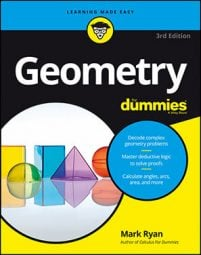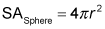##### Geometry For DummiesA sphere doesn't have any flat sides—in fact, its entire surface is one big curve. So finding its surface area must be impossible, right? Actually, it's surprisingly easy. You only need one measurement: its radius.

The radius of a sphere goes from its center to its surface.

Use the following formula for the surface area of a sphere.Because a simple sphere problem would be way too easy to use as an example, let's try something a little more difficult: What's the surface area of a basketball in a box (a cube, of course) if the box has a surface area of 486 square inches?

A cube (or any other ordinary box shape) is a special case of a prism, but you don't need to use the fancy-schmancy prism formula, because the surface area of a cube is simply made up of six congruent squares. Call the length of an edge of the cube s. The area of each side is therefore s2. The cube has six faces, so its surface area is 6s2. Set this equal to the given surface area of 486 square inches and solve for s:

Thus, the edges of the cube are 9 inches, and because the basketball has the same width as the box it comes in, the diameter of the ball is also 9 inches; its radius is half of that, or 4.5 inches. Now you can finish by plugging 4.5 into the surface area formula: